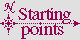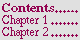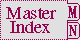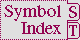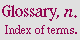#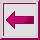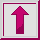## 6.1.8 Examples of Miscellaneous Loop Features

``` (let ((i 0))                     ; no loop keywords are used
(loop (incf i) (if (= i 3) (return i)))) =>  3
(let ((i 0)(j 0))
(tagbody
(loop (incf j 3) (incf i) (if (= i 3) (go exit)))
exit)
j) =>  9
```

In the following example, the variable x is stepped before y is stepped; thus, the value of y reflects the updated value of x:

``` (loop for x from 1 to 10
for y = nil then x
collect (list x y))
=>  ((1 NIL) (2 2) (3 3) (4 4) (5 5) (6 6) (7 7) (8 8) (9 9) (10 10))
```

In this example, x and y are stepped in parallel:

``` (loop for x from 1 to 10
and y = nil then x
collect (list x y))
=>  ((1 NIL) (2 1) (3 2) (4 3) (5 4) (6 5) (7 6) (8 7) (9 8) (10 9))
```

## 6.1.8.1 Examples of clause grouping